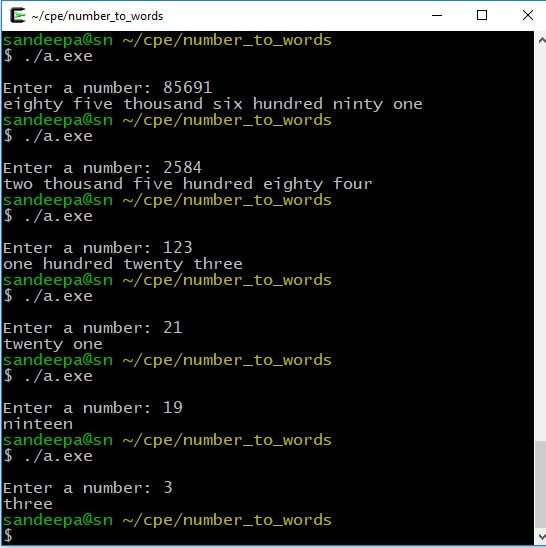# C Program to convert number to words.

### Problem Statement

Write a C Program to convert number to words.
Example:
Input: 4562
Output: four thousand five hundred sixty two

### Solution

The program takes a number between 0 and 99999 as input. First, we count the total number of digits in the number. This is done by successively dividing the number by 10 until we reach 0. Next we go through each digit in the number and decide what to print based on the value of digit at that position. Digit at a particular position can be obtained by dividing the number by a divider. Divider for a given position is pow(10, (position-1)). We start from the left most position and move right as we identify digit at each position and print relevant words to screen.

In the above example, we first divide the number(4562) by 1000 and get 4. We print four thousand and move on to next digit. The next number to work with can be obtained by num % divider. In this case 4562 % 1000 = 562. We divide this number by 100 (divider for position 3) to get 5. So, we print five hundred and move on to next digit. So forth and so on.

#### Special case handling

A value of 1 at position 2 and 5 needs special attention. Take these examples. In 23, when we encounter 2 at position 2, we can be print ‘twenty’ without knowing next digit. It will be taken care of next. But in case of 15, when we encounter 1 in position 2, we can’t print anything yet! It could be any number between eleven and nineteen. So, we have to wait till we see the next digit. To keep track of this, we maintain a flag variable which is set to 1 to indicate such a case.

Read more about C Programming Language . and read the C Programming Language (2nd Edition). by K and R.

### The Program

 #include #include void main() { long num, div, n1; int flag, digit, pos, tot_dig; printf("\nEnter a number: "); scanf("%ld", &num); if(num == 0) { printf("Zeron\n"); exit(0); } if(num > 99999) { printf("please enter a number between 0 and 100000\n\n"); exit(0); } tot_dig = 0; div = 1; n1 = num; while ( n1 > 9 ) { n1 = n1 / 10; div = div * 10; tot_dig++; } tot_dig++; pos = tot_dig; while ( num != 0 ) { digit = num / div; num = num % div; div = div / 10; switch(pos) { case 2: case 5: if ( digit == 1 ) flag = 1; else { flag = 0; switch(digit) { case 2: printf("twenty ");break; case 3: printf("thirty ");break; case 4: printf("forty ");break; case 5: printf("fifty ");break; case 6: printf("sixty ");break; case 7: printf("seventy ");break; case 8: printf("eighty ");break; case 9: printf("ninty "); } } break; case 1: case 4: if (flag == 1) { flag = 0; switch(digit) { case 0 : printf("ten ");break; case 1 : printf("eleven ");break; case 2 : printf("twelve ");break; case 3 : printf("thirteen ");break; case 4 : printf("fourteen ");break; case 5 : printf("fifteen ");break; case 6 : printf("sixteen ");break; case 7 : printf("seventeen ");break; case 8 : printf("eighteen ");break; case 9 : printf("nineteen "); } } else { switch(digit) { case 1 : printf("one ");break; case 2 : printf("two ");break; case 3 : printf("three ");break; case 4 : printf("four ");break; case 5 : printf("five ");break; case 6 : printf("six ");break; case 7 : printf("seven ");break; case 8 : printf("eight ");break; case 9 : printf("nine "); } } if (pos == 4) printf("thousand "); break; case 3: if (digit > 0) { switch(digit) { case 1 : printf("one ");break; case 2 : printf("two ");break; case 3 : printf("three ");break; case 4 : printf("four ");break; case 5 : printf("five ");break; case 6 : printf("six ");break; case 7 : printf("seven ");break; case 8 : printf("eight ");break; case 9 : printf("nine "); } printf("hundred "); } break; } pos--; } if (pos == 4 && flag == 0) printf("thousand"); else if (pos == 4 && flag == 1) printf("ten thousand"); if (pos == 1 && flag == 1) printf("ten "); }
view raw number_to_words.c hosted with ❤ by GitHub

### Sample Output